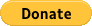Search

# 內部回報率(IRR)Vs NPV

Updated: Sep 9, 2018

IRR 的概念與 NPV 差不多，NPV 是找「淨值」，而 IRR 是找「折舊率」。 若要使 NPV = 0，折舊率要多大呢﹖那折舊率就是 IRR。

Initial Cost - PV(All Cash Flow , IRR) = 0

IRR 越大好 , 或高於目標回報率

NPV = 60/(1+IRR) + 50/(1+IRR)^2 + 20/(1+IRR)^3 = 0

IRR:17%

NPV = 40/(1+IRR) + 40/(1+IRR)^2 + 40/(1+IRR)^3 = 0

IRR﹕9%

0 views

# 最高瀏覽文章

## ​只限持牌顧問登記，成為會員提供保險理財意見給潛在客戶。